# Prime factors

Factor the number 6600 into the product of prime numbers.

x =  6600

### Step-by-step explanation: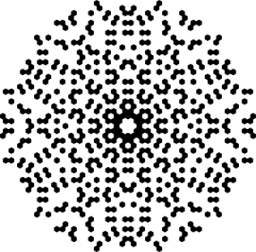Did you find an error or inaccuracy? Feel free to write us. Thank you!## Related math problems and questions:

• Prime factorsWrite 98 as a product of prime factors
• Factors 2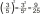A number has 3 identical factors. If 1 of them is 18. Find the number step by step
• Decomposition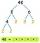Make decomposition using prime numbers of number 155. Result write as prime factors (all, even multiple)
• Prime divisorsFind 2/3 of the sum's ratio and the product of all prime divisors of the number 120.
• Even/odd numbersWhat is the product of 0.97 and the next odd decimal number?
• Amazing numberAn amazing number is name for such even number, the decomposition product of prime numbers has exactly three not necessarily different factors and the sum of all its divisors is equal to twice that number. Find all amazing numbers.
• Find unknown numberWhat is the number between 50 and 55 that is divisible by 2,3,6,9?
• Digits of age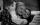The product of the digits of Andrew age as 6 years ago and not equal to 0. Andrew's age is also the youngest possible age with these two conditions. After how many years will the product of the digits of Andrew age again the same as today?
• What fraction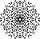What fraction of number 1 to 30 is prime?
• NumberWhat number should be placed instead of the asterisk in number 702*8 to get a number divisible by 6?
• Two numbers and its productThe product of two numbers are 2/3. If on of them is 1/10, what is the other?
• Two friends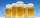Two friends met as a good man perish together for a beer. After recovery the most important topics (politics, women, football ...), one asks: - And how many do you have children? - I have 3 children. - And how many years have? Friend already not want to a
• Count of roots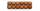How many solutions has equation x. y = 7757 with two unknowns on the set of natural numbers?
• NumbersDetermine the number of all positive integers less than 4183444 if each is divisible by 29, 7, 17. What is its sum?
• Divisible by nineHow many three-digit natural numbers in total are divisible without a remainder by the number 9?
• John SmithJohn Smith is reading a 435-page novel. If he reads the same number of pages per day and the number is a prime number greater than 10, how many pages per day will he read?
• Without 2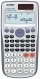Without multipying, tell whether the product 0.644 x 0.25 will be greater than 1 or less than 1? Explane how you know. Then find the product.